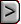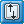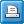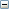﻿ CFP - Conduit Flow Process
Online Guide to MODFLOW

CFP - Conduit Flow ProcessCFP - Conduit Flow ProcessCFP - Conduit Flow ProcessAbbreviation in Name file

CFP

Purpose

The CFP Input File contains most of the information needed to simulate turbulent flow. For example, the location, geometry, and hydraulic properties of conduit flow pipes are held in the CFP Input File, as well as mean void diameters of preferential flow layers.

Documentation

Shoemaker, W.B., Kuniansky, E.L., Birk, S., Bauer, S., and Swain, E.D., 2007, Documentation of a Conduit Flow Process (CFP) for MODFLOW-2005: U.S. Geological Survey Techniques and Methods, Book 6, Chapter A24, 50 p.

Related Packages

 • Conduit Recharge Package (CRCH)
 • Conduit Output Control File (COC)

Supported in

 • MODFLOW-CFP

Other Notes

 • Use convertible layers (LAYCON=2 or 3 in BCF; LAYTYPE = not 0 in LPF; LTHUF = not 0 in HUF2)
 • Use about 10 time steps per stress period to minimize oscillations in turbulent flow.

Input Instructions

Note the data requirements in the CFP Input File change depending upon the desired turbulent flow Mode. Mode 1 (CFPM1) only requires input for conduit flow pipes and nodes, whereas Mode 2 (CFPM2) only requires input for preferential flow layers. Mode 3 (CFPM3) requires input for conduit flow pipes, nodes, and layers. Additionally, the length (LENUNI) and time units (ITMUNI) in the Discretization (DIS) File of MODFLOW-2005 are used within CFP to calculate conduit flow terms, such as gravitational acceleration and viscosity. Thus, if using units of feet and day in the MODFLOW laminar flow model datasets, then units used in the CFP Input File also must be entered in feet and day.

For each simulation, all variables, characters, and arrays in the CFP Input File are read using free format. The required comment lines are dimensioned for 80 characters.

FOR EACH SIMULATION

Data Set 0

[#Text]

Item 0 is a required comment line —“#” must be in column 1.Text
 Text—is a character variable that starts in column 2. Text is generally used to identify input variables and arrays. For example, #Mode for Text labels the underlying integer as the CFP Mode number.

Data Set 1MODE

MODE—is an integer value that activates conduit pipes and (or) layers.

 • If MODE=1, only conduit pipes are active.
 • If MODE=2, only conduit layers are active.
 • If MODE=3, both conduit pipes and layers are active.

 • If MODE=1, datasets 0–29 have to be specified.
 • If MODE=2, only datasets 0, 1, and 30–39 have to be specified.
 • If MODE=3, datasets 0–39 have to be specified

Data sets 2-29 are used only if Mode = 1 or 3.

Data Set 2

[#Text]

Required comment line —“#” must be in column 1.

Data Set 3

[#Text]

Required comment line —“#” must be in column 1.

Data Set 4

NNODES NPIPES NLAYERS (read 1 line)Explanation of variablesNNODES
 NNODES— is an integer value for the total number of nodes in the conduit pipe network. Each node is located at the center of a model cell in plan view.NPIPES
 NPIPES— is an integer value for the total number of pipes in the conduit network.NLAYERS
 NLAYERS— is an integer value for the total number of model layers.

Data Set 5

[#Text]

Required comment line —“#” must be in column 1.

Data Set 6TEMPERATURE
 TEMPERATURE—is a real number in degrees Celsius representing the average temperature of ground water in the conduit pipes.

Data Set 7

[#Text]

Required comment line —“#” must be in column 1.

Repeat data set 8 for each node.

Data Set 8

NO_N  MC  MR  ML  NB1  NB2  NB3  NB4  NB5  NB6  PB1  PB2  PB3  PB4  PB5  PB6Explanation of variablesNO_N
 NO_N (column 1)—is the node number. Nodes are at the center of a model cell and define the connections of the pipe network.MC  MR  ML
 MC, MR, and ML (columns 2 to 4)—are the MOD­FLOW cell column, row, and layer numbers within which node NO_N is located, respectively.NB1  NB2  NB3  NB4  NB5  NB6
 NB1 to NB6 (columns 5 to 10)—are neighbor node numbers, which are connected by pipes to node NO_N (specified in the first column). As many as six adjacent or diagonal neighboring nodes in three dimensions can be listed. Neighbor nodes must be in adjacent cells.  If there are less than six neighbor nodes, insert zeros for the remaining columns. There will always be at least one node connected to the speci­fied node NO_N.PB1  PB2  PB3  PB4  PB5  PB6
 PB1 to PB6 (columns 11 to 16)—are the pipe numbers connected to the node NO_N. Each node should be connected to at least 1 pipe, but can be connected to as many as 6 pipes. Pipe numbers represent the pipe seg­ment between nodes. If there are less than six neighbor­ing pipes connected to the node (NO_N), insert zeros in the remaining columns. Again, a zero in the rest of the columns means there are no more pipe connections.

Data Set 9

[#Text]

Required comment line —“#” must be in column 1.

Data Set 10

[#Text]

Required comment line —“#” must be in column 1.

Data Set 11

[#Text]

Required comment line —“#” must be in column 1.

Data Set 12GEOHEIGHT
 GEOHEIGHT—is the absolute elevation of the pipe nodes. There are two options for entering node elevations. With option 1 the number of lines read is equal to the total number of nodes (NNODES dataset 4). With option 2 only 1 line is read and the first number must equal NNODES in dataset 4. Option 1—NO_N ELEVATION— Each line will con­tain an integer node number (NO_N) and the respective node elevation (ELEVATION) with respect to the user’s model datum. A CFPM1 simulation with 10 nodes, for example, would require 10 lines, each containing an integer node number and the respective node elevation. If the first node number is less than the total number of nodes, this indicates that option 1 has been selected and the CFP program will expect to read in a total number of lines of data equal to the total number of nodes as speci­fied in dataset 4. Option 2—NNODES ELEVATION— only one line is read describing the elevation of each pipe node within finite-difference cells. If the first integer in this section of input equals the total number of nodes as specified in dataset 4, then only one line will be read. This option allows for the CFPM1 to set each node elevation to the vertical center of the encompassing model cell, if ELEVATION is assigned a value of 0.0. Assigning val­ues other than 0.0 for ELEVATION will raise or lower the node elevations a distance equal to ELEVATION above or below the vertical center of the encompassing model cell.

Data Set 13

[#Text]

Required comment line —“#” must be in column 1.

Data Set 14SA_EXCHANGE
 SA_EXCHANGE—is an integer that equals either 0 or 1 If SA_EXCHANGE = 0, the user assigns the pipe con­ductance for each node in the CFP Input File. If SA_EXCHANGE = 1, the user assigns the conduit wall permeability, and the CFP will compute the surface areas of pipes when assembling pipe conductances for groundwater exchange between pipes and porous media.

Data Set 15

[#Text]

Required comment line —“#” must be in column 1.

Data Set 16EPSILON
 EPSILON—is a real number for the convergence criterion of the Newton-Raphson iteration for pipe flow equations. Use a very small number, such as 0.0001.

Data Set 17

[#Text]

Required comment line —“#” must be in column 1.

Data Set 18NITER
 NITER—is an integer number for the maximum number of Newton-Raphson iterations. If convergence cannot be achieved, the program will stop and a warning will be printed in the MODFLOW listing file.

Data Set 19

[#Text]

Required comment line —“#” must be in column 1.

Data Set 20RELAX
 RELAX—is a real number of relaxation that determines the step length of the Newton-Raphson iterations. Changing RELAX to a value slightly less than 1.0 may facilitate convergence of the pipe flow equations.

Data Set 21

[#Text]

Required comment line —“#” must be in column 1.

Data Set 22P_NR
 P_NR—is an integer print flag for Newton Raphson iterations. If P_NR equals 0, results from these iterations are not printed. If P_NR equals 1, results from these iterations are printed, including the MODFLOW itera­tion number, Newton Raphson iteration number, and the maximum node head change.

Data Set 23

[#Text]

Required comment line —“#” must be in column 1.

Data Set 24

[#Text]

Required comment line —“#” must be in column 1.

Repeat data set 25 for each pipe.

Data Set 25

NO_P  DIAMETER  TORTUOSITY  RHEIGHT  LCRITREY_P  TCRITREY_PExplanation of variablesNO_P
 NO_P (column 1)—is the integer pipe number.DIAMETER
 DIAMETER (column 2)—is a real number for the pipe diameter.TORTUOSITY
 TORTUOSITY (column 3)—is a real number for the pipe tortuosity. A value of 1.0 indicates a straight pipe. If a pipe length greater than the straight-line distance between the two nodes of the pipe is required, a tortuos­ity greater than 1 can be used.RHEIGHT
 RHEIGHT (column 4)—is a real number for the internal calculation of roughness and is the mean height of the micro-topography of the conduit  wall.LCRITREY_P
 LCRITREY_P (column 5)—is the lower critical Reyn­olds number (turbulent to laminar).TCRITREY_P
 TCRITREY_P (column 6)—is the upper critical Reyn­olds number (laminar to turbulent).

Data Set 26

[#Text]

Required comment line —“#” must be in column 1.

Repeat data set 27 for each node.

Data Set 27Explanation of variablesNO_N
 NO_N (column 1)—is the integer node number.N_HEAD
 N_HEAD (column 2)—are piezometric heads for nodes with fixed head (positive values) or a flag (-1 negative values less than or equal to -1) that indicates that heads will be calculated during simulation.

Data Set 28

[#Text]

Required comment line —“#” must be in column 1.

Repeat data set 29 for each node

Data Set 29

NO_N K_EXCHANGEExplanation of variablesNO_N
 NO_N (column 1)—is the node number (in increasing order).K_EXCHANGE
 K_EXCHANGE (column 2)—is the pipe conductance (SA_EXCHANGE=0) or conduit wall permeability (SA_EXCHANGE=1).

Data sets 30-39 are used only if Mode = 2 or 3.

Data Set 30

[#Text]

Required comment line —“#” must be in column 1.

Two required text comment lines read only if MODE equals 2 or 3 from Dataset 1. The following variables are required in the CFP Input File for conduit layers. It is important to remember that when MODE equals 2, the following variables are the only variables needed in the CFP Input File. Also, conduit layers must be convertible layers. For example, conduit layers must be specified as LAYCON = 3 in the BCF Package, LAYTYPE not equal 0 in the LPF Package, or LTHUF not equal 0 in the HUF2 Package.

Data Set 31

[#Text]

Required comment line —“#” must be in column 1.

Data Set 32NCL
 NCL—is an integer equal to the total number of conduit layers.

Data Set 33

[#Text]

Required comment line —“#” must be in column 1.

Data Set 34CL
 CL—is a one-dimensional integer array entered on a single line of the CFP Input File. This array holds the MODFLOW layer numbers that are conduit layers.

Data Set 35

[#Text]

Required comment line —“#” must be in column 1.

Data Set 36LTEMP
 LTEMP—is the mean water temperature in degrees Celsius of all conduit layers.

Data Set 37

[#Text]

Required comment line —“#” must be in column 1.

Repeat lines 38 and 39 for each conduit flow layer.

Data Set 38

[#Text]

Required comment line —“#” must be in column 1.

Data Set 39

VOID   LCRITREY_L   TCRITREY_LExplanation of variablesVOID
 VOID—is the mean void diameter. This value is used in calculating the critical head difference from the speci­fied lower and upper critical Reynolds numbers.LCRITREY_L
 LCRITREY_L—is the lower critical Reynolds number for switching from turbulent to laminar flow.TCRITREY_L
 TCRITREY_L—is the upper critical Reynolds number for transitioning from laminar to turbulent flow.